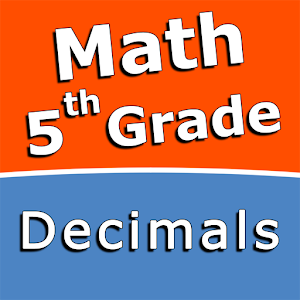Nederlands

We bieden dagelijks GRATIS gelicenseerde Android apps en games aan, die je normaal zou moeten kopen.\$2.99
VERSTREKEN

# Android Giveaway of the Day - Decimals - Fifth grade Math skills

Practice and improve your math skills!
\$2.99 VERSTREKEN
Waarderingscijfer: 0 (0%) 0 (0%)

Deze giveaway is verlopen. Decimals - Fifth grade Math skills is nu beschikbaar op de normale manier.

Intuitive interface powered by handwriting input and a choice of three fun and engaging mini games in addition to a regular math trainer mode make our app stand out from the crowd of generic math learning apps.

With Decimals - Fifth grade Math skills you can practice and improve the following math skills:
- Subtract decimal numbers
- Multiply a decimal by a power of ten
- Multiply a decimal by a one-digit whole number
- Multiply two decimal numbers
- Divide decimals by powers of ten
- Division with decimal quotients
- Divide decimals
- Convert decimals to fractions and mixed numbers
- Convert fractions and mixed numbers to decimals

Choose between four different modes:
- Math Trainer
- Math Shot mini-game
- Math Gravity mini-game
- Math Trick Shot mini-game

Sergey Malugin

Educational

1.0.0

11M

Everyone

4.0 and up

## Reactie op Decimals - Fifth grade Math skills

Thank you for voting!